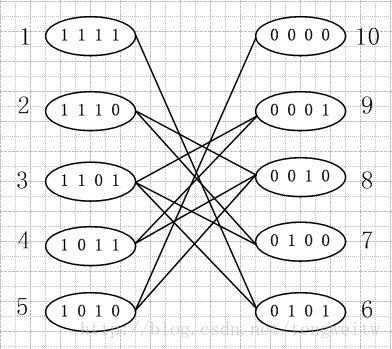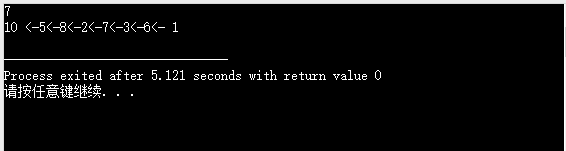• 商人安全过河问题的c++实现。 问题： 三名商人各带一个随从过河，一只小船只能容纳两个人，随从们约定，只要在河的任何一岸，一旦随从人数多于商人人数就杀人越货，但是商人们知道了他们的约定，并且如何过河的大权...c++
• 商人与随从过河，只有一条船，船只能容纳两人，哪一岸随从人数多都属于不安全，如何安排过河才能安全过河
• 请问商人要怎么安全过河？ """ from collections import Counter from random import sample, randint class Solution: """解决问题的方案类""" def __init__(self): """初始化属性""" se
"""
三个商人带了三个随从准备过河，目前只有一条空船(至少需要一人划船)，并且最大承重两人，
这时随从商议在河的任意一岸只要商人的数量少于随从的数量，那随从就会杀人越货，
请问商人要怎么安全过河？
"""

from collections import Counter
from random import sample, randint

class Solution:
"""解决问题的方案类"""

def __init__(self):
"""初始化属性"""
self.left = ['商人'] * 3 + ['随从'] * 3  # 后面用到了Counter，所以这里可以用字符串表示，不用0,1表示，更直观一点
self.left_checkpoint = []  # 左边的存档，用于试错后恢复
self.right = []
self.right_checkpoint = []
self.result = [[]]  # 结果，给个初始值是为了避免out of index的情况，取结果的时候切片即可
self.result_checkpoint = []
self.r_direction = True  # True为右，False为左

def go(self):
"""渡河"""
if self.r_direction:  # 向右渡河
boat = sample(self.left, 2)
for i in boat:
self.left.remove(i)
self.right.append(i)
else:  # 向左渡河
if len(self.right) > 1:  # 这里判断是为了避免sample取的时候越界(从1个里面取2个)
boat = sample(self.right, randint(1, 2))
else:
boat = sample(self.right, 1)
for i in boat:
self.right.remove(i)
self.left.append(i)
return boat

def judge(self):
"""判断"""
if self.left and self.right:
left_counter = Counter(self.left)
right_counter = Counter(self.right)
if (left_counter['商人'] and left_counter['商人'] < left_counter['随从']) or \
(right_counter['商人'] and right_counter['商人'] < right_counter['随从']):
return False
return True

def checkpoint(self):
"""检查点"""
self.left_checkpoint, self.right_checkpoint, self.result_checkpoint = \
self.left.copy(), self.right.copy(), self.result.copy()

def reset(self):
"""读档"""
self.left, self.right, self.result = \
self.left_checkpoint.copy(), self.right_checkpoint.copy(), self.result_checkpoint.copy()

def get_result(self):
"""模拟渡河过程，获取结果"""
while len(self.right) < 6:
self.checkpoint()  # 存档
boat = self.go()  # 渡河
boat.sort()
if self.judge() and boat != self.result[-1]:  # 这里判断是为了避免相同的人来回的情况，以求尽可能少的解
self.r_direction = not self.r_direction  # 调转船头
self.result.append(boat)
else:
self.reset()  # 读档
return self.result[1:]

def main():
"""主函数"""

repeat = 10000  # 重复执行次数
result_set = set()  # 解的集合
solution = Solution()

for _ in range(repeat):
result = solution.get_result()
solution.__init__()

print(f'经{repeat}次执行，共得到{len(result_set)}种不同的结果，结果如下：', end='\n\n')
for result in result_set:
print(result)

if __name__ == '__main__':
main()


结果：
[['随从', '随从'], ['随从'], ['随从', '随从'], ['随从'], ['商人', '商人'], ['商人', '随从'], ['商人', '商人'], ['随从'], ['随从', '随从'], ['商人'], ['商人', '随从']]
[['商人', '随从'], ['商人'], ['随从', '随从'], ['随从'], ['商人', '商人'], ['商人', '随从'], ['商人', '商人'], ['随从'], ['随从', '随从'], ['商人'], ['商人', '随从']]
[['随从', '随从'], ['随从'], ['随从', '随从'], ['随从'], ['商人', '商人'], ['商人', '随从'], ['商人', '商人'], ['随从'], ['随从', '随从'], ['随从'], ['随从', '随从']]
[['商人', '随从'], ['商人'], ['随从', '随从'], ['随从'], ['商人', '商人'], ['商人', '随从'], ['商人', '商人'], ['随从'], ['随从', '随从'], ['随从'], ['随从', '随从']]




展开全文python
• 商人过河问题的Matlab程序，供学习数学建模或者对趣味性数学感兴趣的人参考。
• 商人过河MATLAB程序，通篇只有一个while循环，可实现n个商人，n个仆人情况下的最优解（步骤最少）MATLAB
• 商人过河问题的MATLAB实现，MATLAB源代码。
• 商人怎样安全过河
问题描述

三名商人各带一个随从乘船渡河，一只小船只能容纳两人，由于他们自己划行，随从密约，在和的任一岸，一旦随从的人数比商人多，就杀人越货，但是如何安排乘船的大权安排在商人们的手中，商人们怎样才能安全渡河？

模型假设

我们需要对问题做一些假设：
1. 每个商人和随从都会划船
2. 只有一条船，且每条船上最多只能乘坐两个人
3.  所有商人与随从之间没有矛盾，不会出现两人不愿意坐一条船的现象
4. 船在渡河的过程中不受外界环境的影响

模型构造

记第 k 次过河前此岸的商人数为 xk , 随从数为 yk , k = 1, 2, 3…, xk ,yk = 0, 1, 2, 3

定义状态： 将二维向量 sk = ( xk , yk ) 定义为状态

将安全渡河状态下的状态集合定义为允许状态集合， 记为

S = {(x,y) | x=0,y=0,1,2,3; x=y=1; x=y=2; x=3,y=0,1,2,3}
记第 k 次渡河船上的商人数为 uk ， 随从数为 vk

定义决策： 将二维向量 dk = (uk , vk) 定义为决策

允许决策集合 记作

D = {(u,v) | 1 ≤ u+v ≤ 2, u,v = 0,1,2}
因为小船容量为2，所以船上人员不能超过2，而且至少要有一个人划船，由此得到上式。

由我们定义的状态 sk 和决策 dk ，我们可以发现它们之间是存在联系的：

k 为奇数是表示船由此岸划向彼岸，k 为偶数时表示船由彼岸划回此岸

状态 sk 是随着决策 dk 变化的，规律为：

sk+1 = sk + (-1)kdk
我们把上式称为状态转移律，因此渡河方案可以抽象为如下的多步决策模型：

求决策 dk ∈ D(k = 1,2,…,n) , 使状态 sk ∈ S 按照转移率，初始状态 s1 = (3,3) 经有限步 n 到达状态 sn+1 = (0,0)

到这里，整个数学模型就已经非常清晰了，接下来要做的就是求解模型得出结果。

模型求解

使用dfs就可以，网上找的大牛的C++代码，感觉自己水平还是太菜的 https://www.bbsmax.com/A/Gkz1XLbNdR/

#include <cstdio>
#define maxn 101
int num;//number of bus or fol
int graph[maxn*maxn][maxn*maxn];
int state[maxn][maxn];

//when cross river
int c_bus = {2, 1, 0, 1, 0};
int c_fol = {0, 1, 2, 0, 1};
int b_step[maxn*maxn];
int f_step[maxn*maxn];

bool flag = false;
void DFS(int bus, int fol, int step, int dir)
{
b_step[step] = bus, f_step[step] = fol;
if(bus == 0 && fol == 0)
{
for(int i = 0; i <= step; i++)
{
printf("(%d,%d)", b_step[i], f_step[i]);
if(i != step )
printf(" -> ");
}
printf("\n");
flag = true;
}
int fa = bus * ( num + 1 ) + fol;
for(int i = 0; i < 5; i++)
{
if(dir)
{
int b_next = bus - c_bus[i], f_next = fol - c_fol[i];
if(b_next >= 0 && b_next < num+1 && f_next >= 0 && f_next < num + 1 && state[b_next][f_next])
{
int son = b_next * ( num + 1 ) + f_next;
if(!graph[fa][son] && !graph[son][fa])
{
graph[fa][son] = 1;
graph[son][fa] = 1;
DFS(b_next, f_next, step + 1, !dir);
graph[fa][son] = 0;
graph[fa][son] = 0;
}
}
}
else
{
int b_next = bus + c_bus[i], f_next = fol + c_fol[i];
if(b_next >= 0 && b_next < num + 1 && f_next >= 0 && f_next < num + 1 && state[b_next][f_next])
{
int son = b_next * ( num + 1) + f_next;
if(!graph[fa][son] && !graph[son][fa])
{
graph[fa][son] = 1;
graph[son][fa] = 1;
DFS(b_next, f_next, step + 1, !dir);
graph[fa][son] = 0;
graph[fa][son] = 0;
}
}
}
}
}
int main()
{
scanf("%d",&num);
for(int i = 0; i < num + 1; i++)
{
state[i] = 1;
state[i][num] = 1;
state[i][i] = 1;
}
DFS(num, num, 0, 1);
if(!flag)
printf("they can't cross the river.");
}
展开全文数学建模
• 题意：人带着猫，鸡，米过河，除需要人划船之外，船至多能载猫，鸡，米三者之一，而人不时鸡要吃米，猫要吃鸡，设计一个安全的渡河方案，且渡河次数尽量少以(x, y, z, w)来表示此岸的状态，分别对应着人，猫，鸡，米...
题意：人带着猫，鸡，米过河，除需要人划船之外，船至多能载猫，鸡，米三者之一，而人不时鸡要吃米，猫要吃鸡，设计一个安全的渡河方案，且渡河次数尽量少以(x, y, z, w)来表示此岸的状态，分别对应着人，猫，鸡，米则第k次划船前此岸的允许状态集合为S=｛(1,1,1,1), (1,0,1,1), (1,1,0,1), (1,1,1,0), (1,0,1,0), (0,0,0,0) ,(0,0,0,1), (0,0,1,0), (0,1,0,0), (0,1,0,1)｝;将向量Dk设为允许决策向量,分别表示对应的对象在船上Dk={(1,0,0,0),(1,0,0,1),(1,0,1,0),(1,1,0,0)};Sk+1=Sk+(-1)^k(Dk)便是状态转移方程，实现的话可以用异或要找的便是通过(1,1,1,1)到（0，0，0，0）的最短过程这样实现起来不好实现，刚刚从大佬的博客看到用最短路的方法来做，实现更为简单，但是要先算出来各个状态的转化http://blog.csdn.net/tengweitw/article/details/25296257然后用Dijkstra或者Floyd都可以算#include<bits/stdc++.h>
const int maxn=1e7;
int mp;
int dis,vis,pre;
void init()
{
for(int i=1;i<=10;i++)
{
for(int j=1;j<=10;j++)
{
if(i==j)
mp[i][j]=0;
else
mp[i][j]=maxn;
}
}
mp=mp=mp=mp=1;
mp=mp=mp=mp=1;
mp=mp=mp=mp=1;
mp=mp=mp=mp=1;
mp=mp=mp=mp=1;
}
void Dijkstra(int start,int des)
{
init();
for(int i=1;i<=10;i++)
{
dis[i]=mp[start][i];
}
vis[start]=1;
for(int i=1;i<=10;i++)
{
int min=maxn,min_index=0;
for(int j=1;j<=10;j++)
{
if(min>dis[j] && !vis[j])
{
min=dis[j];
min_index=j;
}
}
vis[min_index]=1;
for(int j=1;j<=10;j++)
{
if(!vis[j] && dis[j]>min+mp[min_index][j])
{
dis[j]=min+mp[min_index][j];
pre[j]=min_index;
}
}
}

printf("%d\n",dis[des]);
printf("%d ",des);
int temp=pre[des];
while(temp!=start && temp!=0)
{
printf("<-%d",temp);
temp=pre[temp];
}
printf("<- %d\n",start);
}
int main()
{
Dijkstra(1,10);
return 0;
}最后得出结果翻译过来就是：1. 人和鸡到对岸  2.人回到此岸 3.人和米到对岸 4.人和鸡到此岸 5.人和猫到对岸 6.人回到此岸 7.人和鸡到对岸
展开全文• 解决了商人过河问题，并且可推广到多种情形，直接用MATLAB运行即可，
• 商人过河问题 1.问题重述 三名商人各带一个随从乘船渡河，一只小船只能容纳二人，由他们自己划行。随从们密约，在河的任一岸，一旦随从的人数比商人多，就杀人越货。但是如何乘船渡河的大权掌握在商人们手中，商人...
商人过河问题
1.问题重述

三名商人各带一个随从乘船渡河，一只小船只能容纳二人，由他们自己划行。随从们密约，在河的任一岸，一旦随从的人数比商人多，就杀人越货。但是如何乘船渡河的大权掌握在商人们手中，商人们怎样才能安全渡河呢?

2.问题分析
该问题是一个多步决策的问题，商人的下一步决定方案依赖于此时的状态，同时商人可以选择的方案有多种可能，因此，要想顺利将商人送达到对岸，需要对每一步进行决策和假设，通过一步步推理，分析出最后的结果
3.模型假设

将商人和随从的数目抽象成具体数值
将河的此岸和彼岸抽象成两种不同的状态，比如说是0和1

4.符号说明
基于递归的方法
假设商人的人数是m，随从的人数是n，船的容量是k。
5.模型的建立和求解
基于递归的方法
记第k次渡河前彼岸（和数学模型（第四版）姜启源 谢金星 叶俊的不一样）的商人数为x，随从数为y，$k=1,2,\dots$，$x=0,1,2,\dots,m$，$y=0,1,2,\dots,n$，将二维向量$s=(x,y)$定义为人员状态，安全渡河条件下的状态集合称为允许人员状态集合，记作$S$
$S=\{(x,y)|x>y \land m-x>n-y\}$
首先需要注意到，此岸的决策恰好与彼岸决策相反，因此，针对此岸和彼岸的状态，需要不同的决策策略。不妨令船在此岸的状态抽象成0，船在彼岸的状态抽象成1。记三元组x, y, d表示彼岸的商人人数、随从人数以及此事船所在的河岸。于是，该事件的起始状态是(0, 0, 0)，即彼岸没有商人和随从，所有人都在此岸，且船在此岸；事件的结束状态是(m, n, 1)，即次岸没有商人和随从，所有人都在彼岸，且船在彼岸。
假设第k次的状态是(x, y, d)，那么他的下一次的状态只能是在$(S, d')$中，所以需要遍历所有可以到达的状态点即可。
6.附录
基于递归的方法的C++代码
#include <iostream>
#include <fstream>
#include <vector>
#include <queue>
#include <stack>
#include <cstring>
#include <memory>

using namespace std;

typedef struct node
{
int x, y, d;
shared_ptr<struct node> prev;// 记录父节点的信息
node(shared_ptr<node> n = nullptr):prev(n) {}
}node;

const int N = 100, M = N * N, mask = 0x1;

int feasible_state[N][N];// 所有可行的位置点
int state[N][N];// 所有可行的状态点 判定状态是否遍历过 (0, 0, 0) 和 (0, 0, 1)是不同的状态
pair<int, int> action[N * N];// 所有可行的决策策略
int m, n, k;// 商人、随从的数量和船的容量
int total_action = 0, all_cnt = 0, ans_cnt = 0, min_lens = 0x3f3f3f3f;// 所有的决策的数目,所有可行方案的数量，最少决策方案的数量，最少方案的决策步数

void init();
void run(shared_ptr<node> now);
bool same(shared_ptr<node> now);
void show_ans();

int main()
{
printf("请输入商人、随从、船容量的信息：<m n k>\n");
scanf("%d%d%d", &m, &n, &k);
init();
show_ans();
system("pause");
return 0;
}

/**
* 初始化所有合法的行动
* 初始化所有合法的状态
* */
void init()
{
// action[]是去向的行动
// action[]是回向的行动
// 使用pair存二维的方向
int method_cnt = 0;
for(int r = 1; r <= k; r++)
for(int j = 0; j <= r; j++)
action[method_cnt] = {j, r - j}, action[method_cnt++] = {-j, j - r};
total_action = max(total_action, method_cnt);

// 所有可达点都是1
for(int j = 0; j <= n; j++)
{
feasible_state[j] = feasible_state[m][j] = feasible_state[j] = feasible_state[m][j] = 1;
for(int i = 0; i <= m - n; i++)
feasible_state[i + j][j] = feasible_state[i + j][j] = 1;
}

// 初试状态是 (0, 0, 0)
// 终点状态是 (m, n, 1)
}

/**
* 核心代码
* 根据所有可行的行动中找到所有到达的合法点进行递归
* */
void run(shared_ptr<node> now)
{
shared_ptr<node> last = make_shared<node>(now->prev), to(new node);

for(int i = 0; i < total_action; i++)
{
to->x = now->x + action[now->d][i].first;
to->y = now->y + action[now->d][i].second;
to->prev = now;

if(to->x < 0 || to->x > m || to->y < 0 || to->y > n) continue;// 出界
if(to->x == m && to->y == n)// 到达终点需要保存这个方案
{
shared_ptr<node> tmp(to);
stack<node> s;
while(tmp != nullptr)
{
s.push(*tmp);
tmp = tmp->prev;
}
all_cnt++;
continue;
}
if(feasible_state[to->x][to->y] == 1 && (last == nullptr || !same(to)))// 到达可行点且没有绕回去
{
run(to);
}
}
}

/**
* 判定是否出现重路
* */
bool same(shared_ptr<node> now)
{
memset(state, 0, sizeof(state));
for(; now != nullptr; now = now->prev)
if(state[now->d][now->x][now->y] == false)
state[now->d][now->x][now->y] = true;
else
return true;
return false;
}

/**
* 展示所有点
* */
void show_ans()
{
{
vector<node> vec;
while(!stk.empty())
{
vec.push_back(stk.top());
stk.pop();
}
}
min_lens = min(min_lens, (int)vec.size());
if(vec.size() == min_lens)
ans_cnt++;

{
{
if(vec.size() == min_lens)
{
for(node n : vec)
answer_file << "(" << n.x << "," << n.y << "," << n.d << ")->";
}
}
}
else
{
printf("一共有 %d 中最短解法\n", ans_cnt);
{
if(vec.size() == min_lens)
{
for(node n : vec)
printf("(%d,%d,%d)->", n.x, n.y, n.d);
printf("\n");
}
}
}
}


参考 商人过河问题


展开全文• 在河的任何一边，只要仆人的数量超过商人的数量，仆人就会联合起来将商人杀死并抢夺其财物，问商人应如何设计过河顺序才能让所有人都安全地过到河的另一边。 详细过程参见《数学模型》第四版（姜启源） #...c++ 算法 dfs
• ## 商人过河问题(n个)-Matlab

万次阅读 多人点赞 2018-06-11 23:58:25
商人过河问题：n名商人各带一名随从过河，一只小船只能容纳z个人，随从们约定，只要在河的任何一岸，一旦随从人数多于商人人数就杀人越货，但是商人们知道了他们的约定，并且如何过河的大权掌握在商人们手中，商人们...Matlab
• (回溯算法)商人怎样安全过河 (本文属原创,转载请注名作者!author:wcnpepyu) 链接：http://blog.csdn.net/wengjiaxiang/article/details/1523696 过河问题： 有三个商人和三个仆人过河，只有一条能装下两个人的...
• ​ 将过河问题抽象为一个数学问题，安全渡河即为一个多步决策问题，在安全的前提下，每一步都考虑船上的商人与随从人数情况。 ​ 决策问题通常从考虑状态，决策，状态转移方程入手。 状态 ​ 设sk=(xk,yk)s_k=(x_...
• 过河问题： 　 　有三个商人和三个仆人过河，只有一条能装下两个人的船，在河的任何一岸上，如果仆人的人数大于商人的人数，那么该岸上的商人就会有危险。你能不能找出一种安全的渡河方法呢？ 　 　过河问题是一个...
• '''三商人三仆人过河问题，有一条船，最多只允许乘2人，要求商人数不少于仆人数， 设计过河方案''' '''基于状态空间搜索，深度优先搜索'''python 状态空间搜索 搜索
• 商人们怎样才能安全过河 -名商人 - 问题分析多步决策过.程 XXX 3名随从 决策~每一步(此岸到彼岸或彼岸到此岸)船上的人员 要求~在安全的前提下(两岸的随从数不比商人多,经有限步使全体人员过河 建立模型 xk~第 xk~第...
• 商人们怎样安全过河随从们密约,在河的任一岸,一旦随从的人数比商人多,就杀人越货.但是乘船渡河的方案由商人决定.商人们怎样才能安全过河?问题分析：多步决策过程决策~每一步(此岸到彼岸或彼岸到此岸)船上的人员要求~...java hashmap string path class
• 如果有n名商人，有n名随从，如何建模？设计求解算法？是否n为任意值均有解？ 问题解答： 假设第k次渡河前， xk表示此岸的商人数， yk为随从数。 S表示安全渡河条件下的状态集合： S={(X,Y)|X=0，Y=0,1,2,3...N:X...
• 对于每一步的决策，可以选择一定数量的商人和仆人上船，然后在河的左岸和右岸之间进行摆渡，并且保证每一次摆渡都不能使得商人被杀死（当然，如果不存在一种安全过河的方案，那么商人必死） 当n=3，r=2时，船的左岸......